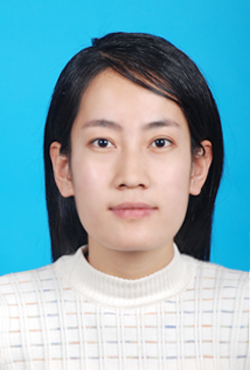发布时间: 2013-05-10 　　浏览次数: 5326

 姓　　名 高广花 性　　别 女出生年月 1985年11月 学历学位 研究生学历,理学博士学位 职　　称 副教授 部　　门 理学院工程数学教学中心 导师类别 硕导 指导专业 计算数学 办公地点 仙林教2-500(1) 办公电话 85866605 电邮地址 gaogh@njupt.edu.cn 个人主页 点击查看更多信息 主授课程 偏微分方程数值解法、线性代数与解析几何、概率统计与随机过程等 社会兼职 研究方向 偏微分方程数值解 个人简历 2002.9-2006.7: 天津师范大学数学科学学院2006.9-2009.7: 天津师范大学数学科学学院2009.9-2012.6: 东南大学数学系2012.6至今：南京邮电大学理学院工作2014.3-2014.9: 澳门大学科技学院博士后研究 主要成果 从事偏微分方程数值解的研究工作，现主要集中于分数阶偏微分方程数值解的研究，取得了一些研究成果。主持完成国家级项目2项和省部级项目1项，主持在研省部级项目1项，参与完成项目若干项。合作出版专著三部，发表SCI论文近30篇。 研究项目 1. 求解四阶时间多项分数阶扩散波方程的差分算法研究(BK20191375)，江苏省自然科学基金面上项目，2019.07-2022.06 (主持，在研)2. 数值求解分数阶偏微分方程的高精度快速算法研究(11401319)，国家自然科学青年基金项目，2015.01-2017.12 (主持，已结题)3. 分数阶偏微分方程的高精度有限体积元方法研究(11326225)， 国家自然科学数学天元基金专项基金项目, 2014.01-2014.12 (主持, 已结题)3. 时间分数阶偏微分方程的高精度差分算法设计及理论分析(BK20130860) , 江苏省自然科学青年基金项目, 2013.07-2016.06(主持，已结题)4. 求解分数阶偏微分方程的高精度算法研究(NY213051)，南京邮电大学校引进人才科研启动基金项目，2013.07-2016.06 (主持，已结题)5. 异质多智能体系统的异步采样协调动力学分析( BK20181387)，江苏省自然科学基金面上项目，2018.07-2021.06 (参与，在研)6. 可分非凸优化的分解算法及其在图像分割中的应用研究(11501301) , 国家自然科学青年基金, 2016.01-2018.12 (参与, 已结题)7. 网络化异质多智能体系统的协调动力学分析与控制(61304169) , 国家自然科学青年基金, 2014.01-2016.12 (参与, 已结题)8. 空间分数阶偏微分方程高精度快速算法的研究(11271068)，国家自然科学面上项目，2013.01-2016.12 (参与, 已结题) 荣誉奖励 2019年度荣获南京邮电大学科学技术奖一等奖；2018年度荣获南京市第12届自然科学优秀学术论文优秀奖；2016年度入选南京邮电大学1311人才计划“鼎新学者”；2015年度荣获南京市第11届自然科学优秀学术论文三等奖。 论著代表作   《分数阶微分方程的有限差分方法（第二版）》，孙志忠，高广花编，科学出版社，北京，2021年1月。（信息与计算科学丛书第87本）  Fractional Differential Equations-Finite Difference Methods. Zhi-zhong Sun, Guang-hua Gao. DE GRUYTER/Science Press Beijing, 2020.08.  《分数阶微分方程的有限差分方法》，孙志忠，高广花编，科学出版社，北京，2015年8月。（信息与计算科学丛书第70本）  Guang-hua Gao, Rui Tang, Qian Yang, A compact finite difference scheme for the fourth-order time multi-term fractional sub-diffusion equations with the first Dirichlet boundary conditions, International Journal of Numerical Analysis and Modeling, 18 (2021), pp. 100-119. (SCI) Guang-hua Gao, Qian Yang, Fast evaluation of linear combinations of Caputo fractional derivatives and its applications to multi-term time-fractional sub-diffusion equations, Numerical Mathematics: Theory, Methods and Applications, 13 (2020), pp. 433-451.(SCI)  Guang-hua Gao, Rui Liu,A compact difference scheme for fourth-order multi-term fractional wave equations and maximum error estimates, East Asian Journal on Applied Mathematics, 9 (2019), pp. 703-722. (SCI)  Guang-hua Gao, Anatoly A. Alikhanov, Zhi-zhong Sun, The temporal second order difference schemes based on the interpolation approximation for solving the time multi-term and distributed-order fractional sub-diffusion equations, Journal of Scientific Computing, 73 (2017), pp. 93-121.  (SCI)  Guang-hua Gao, Zhi-zhong Sun, Two difference schemes for solving the one-dimensional time distributed-order fractional wave equations, Numerical Algorithms, 74 (2017), pp. 675-697. (SCI)  Guang-hua Gao, Zhi-zhong Sun, Two alternating direction implicit difference schemes for solving the two-dimensional time distributed-order wave equations, Journal of Scientific Computing, 69 (2016), pp. 506-531. (SCI)   Guang-hua Gao, Zhi-zhong Sun, Two alternating direction implicit difference schemes for two-dimensional distributed-order fractional diffusion equations, Journal of Scientific Computing,  66 (2016), pp. 1281-1312. (SCI)   Guang-hua Gao, Zhi-zhong Sun, Two unconditionally stable and convergent difference schemes with the extrapolation method for the one-dimensional distributed-order differential equations, Numerical Methods for Partial Differential Equations, 32 (2016) , pp. 591-615. (SCI)   Guang-hua Gao, Zhi-zhong Sun, Two alternating direction implicit difference schemes with the extrapolation method for the two-dimensional distributed-order differential equations, Computers and Mathematics with Applications, 69 (2015)  pp. 926-948.  (SCI)   Guang-hua Gao, Hai-wei Sun, Zhi-zhong Sun, Some high-order difference schemes for the distributed-order differential equations, Journal of Computational Physics,  298 (2015) pp. 337-359. (SCI)   Guang-hua Gao, Hai-wei Sun, Zhi-zhong Sun, Stability and convergence of finite difference schemes for a class of time-fractional sub-diffusion equations based on certain superconvergence, Journal of Computational Physics, 280(2015)  pp. 510-528. (SCI)   Guang-hua Gao, Hai-wei Sun, Three-point combined compact difference schemes for time-fractional advection-diffusion equations with smooth solutions, Journal of Computational Physics, 298 (2015) pp.520-538. (SCI)  Guang-hua Gao, Hai-wei Sun, Three-point combined compact alternating direction implicit difference schemes for two-dimensional time-fractional advection-diffusion equations, Communications in Computational Physics, 17(2015) pp. 487-509. (SCI)    Guang-hua Gao, Zhi-zhong Sun, Hong-wei Zhang, A new fractional numerical differentiation formula to approximate the Caputo fractional derivative and its applications, Journal  of  Computational Physics, 259 (2014) pp. 33-50.  (SCI)  Guang-hua Gao, Zhizhong Sun, The finite difference approximation for a class of fractional sub-diffusion equations on a space unbounded domain, Journal of Computational Physics, 236, 443-460, 2013. (SCI)   Guang-hua Gao, Zhi-zhong Sun, Compact difference schemes for heat equation with Neumann boundary conditions (II), Numerical Methods for Partial Differential Equations, 29 (2013) pp. 1459-1486. (SCI)   Guang-hua Gao, Zhi-zhong Sun, Ya-nan Zhang, A finite difference scheme for fractional sub-diffusion equations on an unbounded domain using artificial boundary conditions, Journal of Computational Physics, 231 (2012),  pp. 2865-2879. (SCI)   Guang-hua Gao, Zhi-zhong Sun, Finite difference approach for the initial-boundary value problem of the fractional Klein-Kramers equation in phase space, Central  European Journal of  Mathematics, 10 (2012) pp. 101-115. (SCI)   Guang-hua Gao, Zhi-zhong Sun, A compact finite difference scheme for the fractional sub-diffusion equations, Journal of Computational Physics，230 (2011), pp. 586-595. (SCI) Guang-hua Gao, Tong-ke Wang, Cubic superconvergent finite volume element method for one-dimensional elliptic and parabolic equations, Journal of Computational and Applied Mathematics, 233 (2010), pp. 2285-2301. (SCI)   Hong Sun, Zhi-zhong Sun, Guang-hua Gao, Some temporal second order difference schemes for fractional wave equations, Numerical Methods for Partial Differential Equations, 32 (2016), pp. 970-1001. (SCI)   Hong Sun, Zhi-zhong Sun, Guang-hua Gao, Some high order difference schemes for the space and time fractional Bloch-Torrey equations, Appliced Mathematics and Computation, 281 (2016), pp. 356-380. (SCI)   Tong-ke Wang, Na Li, Guang-hua Gao, The asymptotic expansion and extrapolation of trapezoidal rule for integrals with fractional order singularities, International Journal of Computer Mathematics, 92 (2015) pp. 579-590. (SCI)   Zhi-fang Liu, Tong-ke Wang ,Guang-hua Gao, A local fractional Taylor expansion and its computation for insufficiently smooth functions, East Asian Journal on Applied Mathematics, 5 (2015) pp. 176-191. (SCI)   Zhifang Liu, Tongke Wang, Guang-hua Gao, A local fractional Taylor expansion and its computation for insufficiently smooth functions, East Asian Journal on Applied Mathematics, 5 (2015) pp. 176-191.   Ri Du, Zhi-zhong Sun, Guang-hua Gao, A second-order linearized three-level backward Euler scheme for a class of nonlinear expitaxial growth model, International Journal of Computer Mathematics, 92 (2015), pp. 2290-2309 (SCI)   Jin-cheng Ren, Guang-hua Gao, Efficient and stable numerical methods for the two-dimensional fractional Cattaneo equation, Numerical Algorithms, 69 (2015), pp. 795-818 (SCI)   Hai-yan Cao, Zhi-zhong Sun, Guang-hua Gao, A three-level linearized finite difference scheme for the Camassa-Holm equation, Numerical Methods for Partial Differential Equations, 30 (2014) pp.451-471. (SCI)   Peng Mao, Jing Chen, Rongqing Xu, Guozhi Xie, Yuanjian Liu, Guang-hua Gao, Shan Wu, Self-assembled silver nanoparticles: correlation between structural and surface Plasmon resonance properties, Applied Physics A, 117 （2014）pp. 1067-1073. (SCI)Rongqing Xu, Yunqing Lu, Chunhui Jiang, Jing Chen, Peng Mao, Guang-hua Gao, Labao Zhang, Shan Wu, Facile fabrication of three-dimensional Graphene foam/poly(dimethylsiloxane) composites and their potential application as strain sensor, Applied Material & Interfaces, 6 (2014) pp.13455-13460. (SCI)刘蕊，高广花，袁安安，求解一类多项时间四阶时间分数阶慢扩散系统的有限差分格式，宁夏大学学报（自然科学版），Vol. 38, No. 2 (2017) pp. 1-10.王星，高广花，王同科，半线性抛物问题的一类三次有限体积元方法，辽宁工程技术大学学报（自然科学版），Vol. 34, No. 2 (2015) pp. 281-284.李娟，高广花，求解Fisher-Kolmogorov方程的三层线性化紧差分格式，西南民族大学学报（自然科学版），Vol. 41, No. 5 (2015) pp. 634-639.高广花，王同科，一类拟线性神经传播方程的紧LOD差分格式，天津师范大学学报（自然科学版），Vol. 29, No. 1 (2009) pp. 1-6.高广花，王同科，两点边值问题基于三次样条插值的高精度有限体积元方法，山东大学学报（理学版），Vol. 44, No. 2 (2009) pp. 45-51. 联系地址 江苏省 南京市亚东新城区文苑路9号, 南京邮电大学 理学院 邮政编码 210023